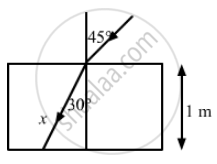Advertisement Remove all ads

# A Light Ray Falling at an Angle of 45° with the Surface of a Clean Slab of Ice of Thickness 1.00 M is Refracted into It at an Angle of 30° - Physics

Sum

A light ray falling at an angle of 45° with the surface of a clean slab of ice of thickness 1.00 m is refracted into it at an angle of 30°. Calculate the time taken by the light rays to cross the slab. Speed of light in vacuum = 3 × 108 m s−1.

Advertisement Remove all ads

#### Solution

Given,
Angle of incidence, i = 45°
Angle of refraction, r = 30°
Using Snell's law,
$\frac{\sin i}{\sin r} = \frac{3 \times {10}^8}{v}$
$= \frac{\sin 45^\circ}{\sin 30^\circ } = \frac{\left( \frac{1}{\sqrt{2}} \right)}{\left( \frac{1}{2} \right)}$
$= \frac{2}{\sqrt{2}} = \sqrt{2}$

$So,$

$v = \frac{3 \times {10}^8}{\sqrt{2}} m/s$
Let x be the distance travelled by light in the slab.
Now,
$x = \frac{1 m}{\cos 30^\circ} = \frac{2}{\sqrt{3}} m$
We know:
Time taken
$= \frac{Distance}{Speed}$
$= \frac{2}{\sqrt{3}} \times \frac{\sqrt{2}}{3 \times {10}^8}$
= 0.54 × 10−8
= 5.4 × 10−9 sConcept: Ray Optics - Mirror Formula
Is there an error in this question or solution?
Advertisement Remove all ads

#### APPEARS IN

HC Verma Class 11, 12 Concepts of Physics 1
Chapter 18 Geometrical Optics
Q 14 | Page 413
Advertisement Remove all ads

#### Video TutorialsVIEW ALL 

Advertisement Remove all ads
Share
Notifications

View all notifications

Forgot password?